Energy & Mining Engineering Front 2017

Next Previous

Image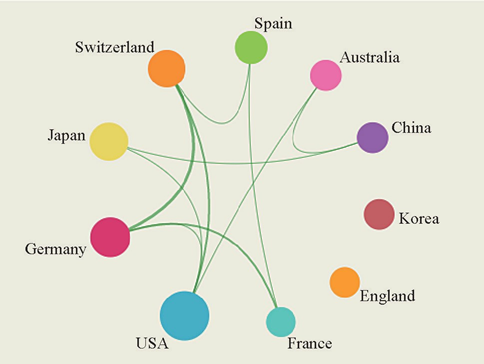Figure 1.2.1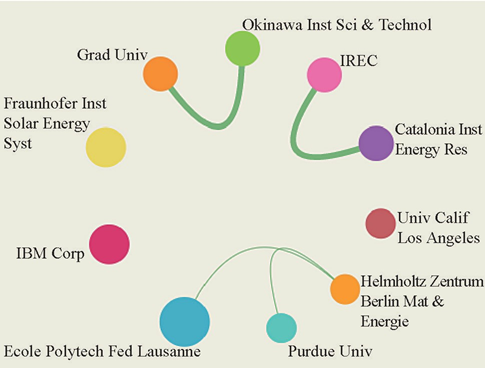Figure 1.2.2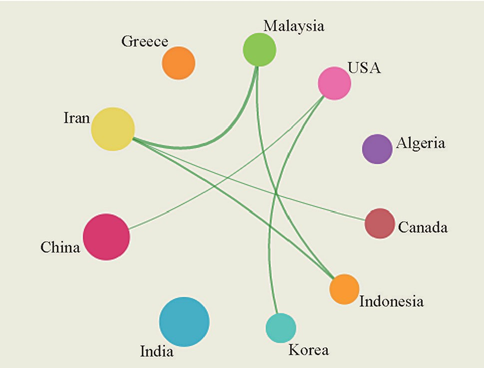Figure 1.2.3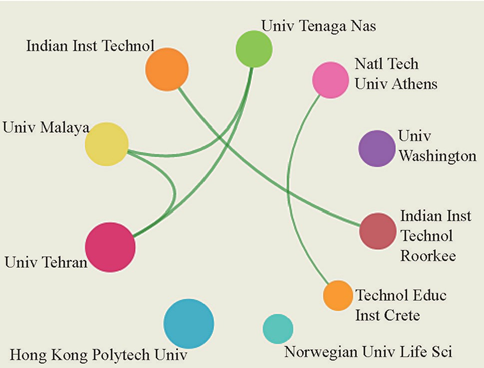Figure 1.2.4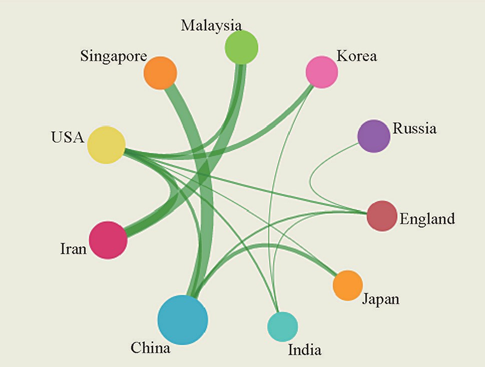Figure 1.2.5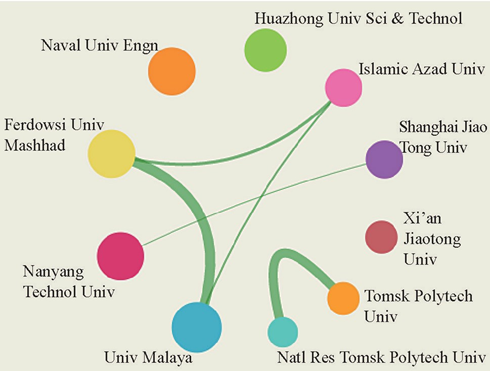Figure 1.2.6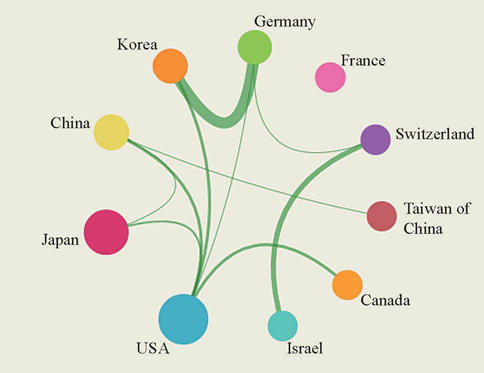Figure 2.2.1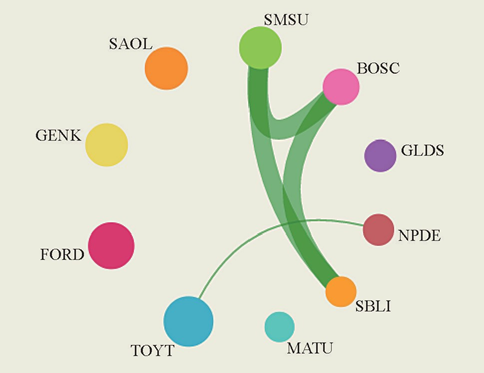Figure 2.2.2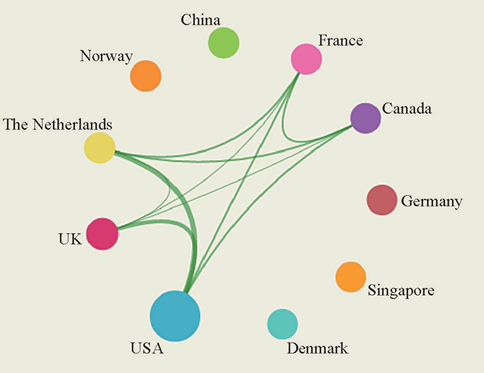Figure 2.2.3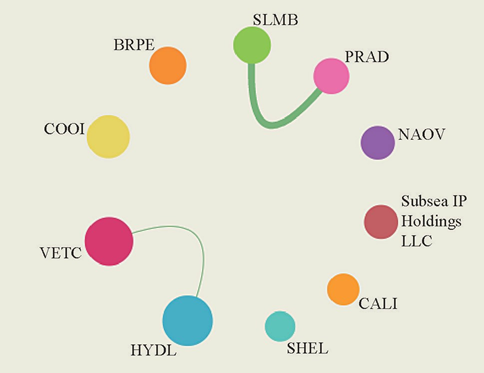Figure 2.2.4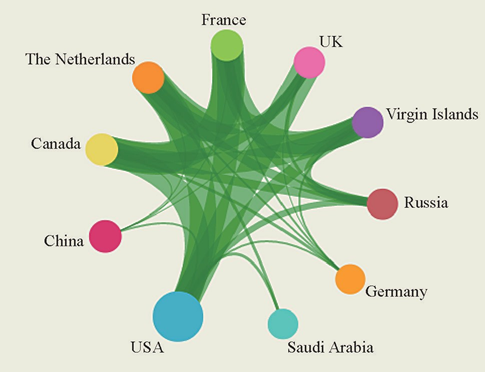Figure 2.2.5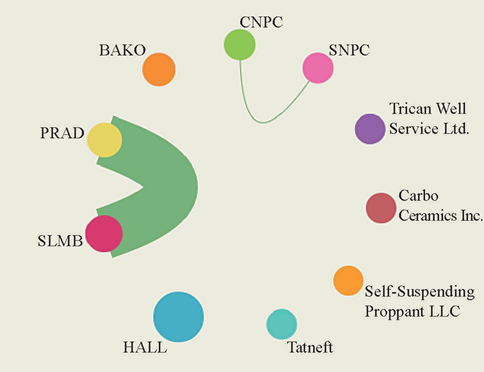Figure 2.2.6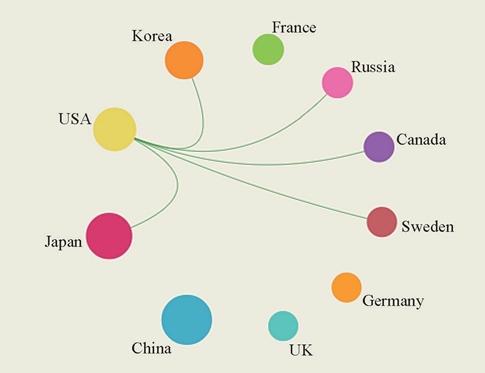Figure 2.2.7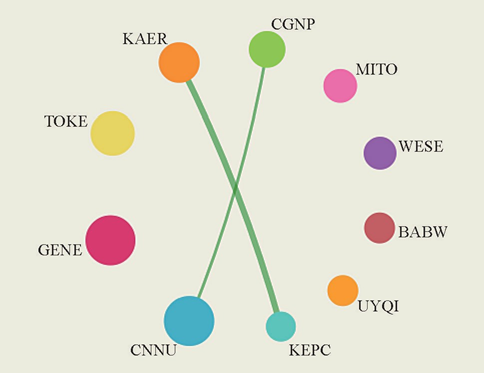Figure 2.2.8

Related Research